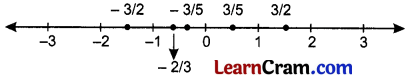# DAV Class 7 Maths Chapter 1 Brain Teasers Solutions

The DAV Class 7 Maths Book Solutions Pdf and DAV Class 7 Maths Chapter 1 Brain Teasers Solutions of Rational Numbers offer comprehensive answers to textbook questions.

## DAV Class 7 Maths Ch 1 Brain Teasers Solutions

Question 1.
A. Tick (✓) the correct option:
(i) The value ofx such that $$\frac{-3}{8}$$ and $$\frac{x}{-24}$$ are equivalent rational numbers is-
(a) 64
(b) -64
(c) -9
(d) 9
$$\frac{-3}{8}, \frac{x}{-24}$$ will be equivalent if -3 × -24 = x × 8
⇒ x = $$\frac{-3 \times-24}{8}$$
= -3 × -3 = 9
Therefore, x = 9
Hence, (d) is the correct option.

(ii) Which of the following is a negative rational number?
(a) $$\frac{-15}{-4}$$
(b) 0
(c) $$\frac{-5}{7}$$
(d) $$\frac{4}{9}$$
$$\frac{-5}{7}$$ is a negative rational number as the signs of the numerator and the denominator are different.
Hence, (c) is the correct option.

(iii) In the given number line, which of the following rational numbers does the point M represent?(a) $$\frac{2}{8}$$
(b) $$\frac{3}{5}$$
(c) $$\frac{2}{3}$$
(d) $$\frac{12}{5}$$
The point M is represented by fraction $$\frac{12}{20}$$. Now, HCF of 12 and 20 is 4.
∴ $$\frac{12 \div 4}{20 \div 4}=\frac{3}{5}$$ which is the standard form.
Hence, (b) is the correct answer.

(iv) Which is the greatest rational number out of $$\frac{5}{-11}, \frac{-5}{12}, \frac{5}{-17}$$?
(a) $$\frac{5}{-11}$$
(b) $$\frac{-5}{12}$$
(c) $$\frac{5}{-17}$$
(d) cannot be compared
First, write $$\frac{5}{-11}, \frac{-5}{12}, \frac{5}{-17}$$ in standard form, i.e., $$\frac{-5}{11}, \frac{-5}{12}, \frac{-5}{17}$$
Now, find the productsThe products are -5 × 12 = -60 and -5 × 11= -55.
Since, -60 < -55, therefore, $$\frac{-5}{11}<\frac{-5}{12}$$
Now, find the productsThe products are -5 × 17 = -85 and -5 × 12 = -60.
Since -85 < -60, therefore, $$\frac{-5}{12}<\frac{-5}{17}$$.
Therefore, $$\frac{-5}{17}$$ is the greatest rational number.
Hence, (c) is the correct option.

(v) Which of the following rational numbers is the smalles?
(a) $$\left|\frac{7}{11}\right|$$
(b) $$\left|\frac{-8}{11}\right|$$
(c) $$\left|\frac{-2}{11}\right|$$
(d) $$\left|\frac{-9}{-11}\right|$$
Absolute values of the given rational numbers are
$$\left|\frac{7}{11}\right|=\frac{7}{11},\left|\frac{-8}{11}\right|=\frac{8}{11},\left|\frac{-2}{11}\right|=\frac{2}{11}$$ and $$\left|\frac{-9}{-11}\right|=\frac{9}{11}$$

Now, the rational numbers $$\frac{7}{11}, \frac{8}{11}, \frac{2}{11}$$ and $$\frac{9}{11}$$ have same denominator, therefore, smaller the numerator, smaller will be the rational number.

Since 2 < 7 < 8 < 9, therefore, $$\frac{2}{11}<\frac{7}{11}<\frac{8}{11}<\frac{9}{11}$$
$$\left|\frac{-2}{11}\right|<\left|\frac{7}{11}\right|<\left|\frac{-8}{11}\right|<\left|\frac{-9}{-11}\right|$$

Therefore, $$\left|\frac{-2}{11}\right|$$ is the smallest rational number.
Hence, (c) is the correct answer(i) Find the average of the rational numbers $$\frac{4}{5}, \frac{2}{3}, \frac{5}{6}$$.
(ii) How will you write $$\frac{12}{-18}$$ in th e standard form?
(iii) How many rational numbers are there between any two rational numbers ?
(iv) On the number line, the rational number $$\frac{-5}{-7}$$ lies on which side of zero?
(v) Express $$\frac{-7}{-8}$$ as a rational number with denominator 40.
(i) We Know Average = $$\frac{\text { Sum of all observations }}{\text { Number of observations }}$$
Therefore, average of the rational numbers $$\frac{4}{5}, \frac{2}{3}, \frac{5}{6}$$ is given by
Average = $$\frac{\left(\frac{4}{5}+\frac{2}{3}+\frac{5}{6}\right)}{3}$$
= $$\frac{\left(\frac{24+20+25}{30}\right)}{3}=\frac{\left(\frac{69}{30}\right)}{3}$$
= $$\frac{69}{30 \times 3}$$
= $$\frac{23}{30}$$

(ii) Step 1: Convert the denominator into positive by multiplying numerator and denominator by -1.
i.e., $$\frac{(12) \times(-1)}{(-18) \times(-1)}=\frac{-12}{18}$$

Step 2: Find HCF of 12 and 18, which is 6 in this case, and divide numerator and denominator by it.
i.e., $$\frac{-12 \div 6}{18 \div 6}=\frac{-2}{3}$$ which is the standard form.

(iii) Infinitely many rational numbers lie between any two rational numbers.

(iv) First, convert the denominator into positive by multiplying and denominator by -1.
∴ $$\frac{-5 \times(-1)}{-7 \times(-1)}=\frac{5}{7}$$
$$\frac{5}{7}$$ > 0, therefore, $$\frac{5}{7}$$ lies to the left of the zero.

(v) To get denominator 40, we must multiply the given denominator -8 by -5.
i.e., $$\frac{(-7) \times(-5)}{(-8) \times(-5)}=\frac{35}{40}$$
Hence, the required rational number is $$\frac{35}{40}$$.

Question 2.
State whether the following statements are true. If not, then give an example in support of your answer.
(i) If $$\frac{p}{q}>\frac{r}{s}$$ then $$\left|\frac{p}{q}\right|>\left|\frac{r}{s}\right|$$
If $$\frac{p}{q}>\frac{r}{s}$$ is true if $$\frac{p}{q}$$ and $$\frac{r}{s}$$ are both positive rational numbers.

But $$\left|\frac{p}{q}\right|>\left|\frac{r}{s}\right|$$ means that $$\frac{p}{q}>\frac{r}{s}$$ and $$\frac{-p}{q}>\frac{-r}{s}=\frac{p}{q}<\frac{r}{s}$$
$$\left|\frac{p}{q}\right|>\left|\frac{r}{s}\right|$$ is not true statements

(ii) If |x| = |y| then x = y
If |x| = |y | ∴ x = y and -x = -y
So it is true.

(iii) $$\frac{p}{q}$$ is a non-zero rational number in standard form. It is necessary that rational number $$\frac{p}{q}$$ will be in standard form.
No it is not necessary

Question 3.
Represent 5$$\frac{1}{3}$$ and $$\frac{-29}{4}$$ on a number line.
5$$\frac{1}{3}$$ = $$\frac{16}{3}$$Question 4.
Arrange the following rational numbers in descending order
$$\frac{-3}{10}, \frac{-7}{-5}, \frac{9}{-15}, \frac{18}{30}$$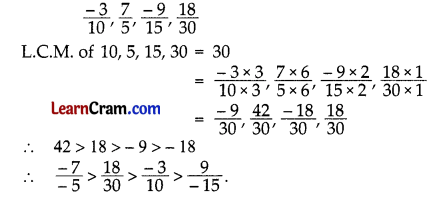Question 5.
On a number line, what is the length of the line segment joining.
(i) 3 and -3
3 – (-3) = 6 units

(ii) $$\frac{1}{2}$$ and –$$\frac{1}{2}$$
$$\frac{1}{2}-\left(-\frac{1}{2}\right)=\frac{1}{2}+\frac{1}{2}$$ = 1 unit

(iii) $$\frac{1}{2}$$ and 2$$\frac{1}{2}$$
$$\frac{1}{2}$$ and $$\frac{5}{2}=\frac{5}{2}-\frac{1}{2}$$ = 2 units

(iv) –$$\frac{1}{2}$$, -2$$\frac{1}{2}$$
$$\frac{-1}{2}$$ and $$\frac{-5}{2}=\frac{-1}{2}-\left(\frac{-5}{2}\right)=\frac{-1}{2}+\frac{5}{2}$$
= 2 units

Question 6.
Find the value of x is each case.
(i) $$\frac{23}{x}=\frac{2}{-8}$$
$$\frac{23}{x}=\frac{2}{-8}$$
⇒ 2 × x = 23x – 8
⇒ x = $$\frac{23 x-8}{2}$$
∴ x = -92

(ii) $$\frac{x}{9}=\frac{19}{3}$$
$$\frac{x}{9}=\frac{19}{3}$$
⇒ 3 × x = 19 × 9
⇒ x = $$\frac{19 \times 9}{3}$$ = 57
∴ x = 57

(iii) $$\frac{15}{-x}=\frac{1}{-7}$$
$$\frac{15}{-x}=\frac{1}{-7}$$
⇒ -x = -105
∴ x = 105

Question 7.
(i) $$\frac{-5}{7}, \frac{9}{-13}$$
$$\frac{-5}{7}, \frac{9}{-13}$$ = -5 × -13, 7 × 9 = 65 > 63
∴ $$\frac{-5}{7}>\frac{9}{-13}$$

(ii) $$\frac{-4}{9}, \frac{-3}{7}$$
$$\frac{-4}{9}, \frac{-3}{7}$$
= -4 × 7, -3 × 9
= -28 < – 27

(iii) $$\frac{-3}{-5}, \frac{12}{20}$$
$$\frac{-3}{-5}, \frac{12}{20}$$
= -3 × 20, 12 × -5
= -60 = -60
∴ $$\frac{-3}{-5}=\frac{12}{20}$$

(iv) $$\left|\frac{-4}{5}\right|,\left|\frac{-5}{4}\right|$$
$$\left|\frac{-4}{5}\right|,\left|\frac{-5}{4}\right|$$ =  = 4 × 4, 5 × 5
= 16 < 25
∴ $$\left|\frac{-4}{5}\right|<\left|\frac{-5}{4}\right|$$

(v) $$\left|\frac{5}{7}\right|,\left|\frac{-15}{21}\right|$$
$$\left|\frac{5}{7}\right|,\left|\frac{-15}{21}\right|=\frac{5}{7}, \frac{15}{21},=\frac{5}{7}=\frac{5}{7}$$
∴ $$\left|\frac{5}{7}\right|=\left|\frac{-15}{21}\right|$$

(vi) $$\left|\frac{-8}{-9}\right|,\left|\frac{-3}{9}\right|$$
$$\left|\frac{-8}{-9}\right|,\left|\frac{-3}{9}\right|=\frac{8}{9}, \frac{3}{9}$$ = 8 × 9 > 9 × 3 = 72 > 27
∴ $$\left|\frac{-8}{-9}\right|>\left|\frac{-3}{9}\right|$$Question 8.
Fill in the following blanks.
(i)$$\frac{3}{5}=\frac{138}{x}$$
⇒ 3x = 5 × 138
∴ x = $$\frac{5 \times 138}{3}$$ = 230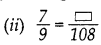$$\frac{7}{9}=\frac{y}{108}$$
⇒ 9 × y = 7 × 108
∴ y = $$\frac{7 \times 108}{9}$$ = 84$$\frac{z}{-15}=\frac{48}{90}$$
⇒ 9 × z = -15 × 48
∴ z = $$\frac{-15 \times 48}{90}$$
∴ z = -8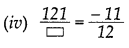$$\frac{121}{v}=\frac{-11}{12}$$
⇒ -11v = 121 × 12
∴ v = $$\frac{121 \times 12}{-11}$$
∴ v = -132

## DAV Class 7 Maths Chapter 1 HOTS

Question 1.
The points P, Q, R, S, T, U, A and B are on the number line representing integers such that TR = RS = SU and AP = PQ = QB
Locate and write the rational numbers represented by points P, Q, R, and S.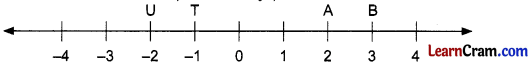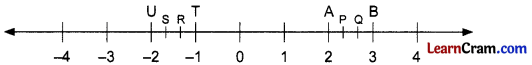As AP = PQ = QB so it means that line-segment AB is divided into three equal parts.
Now, point P represents 1$$\frac{1}{3}$$ i.e., $$\frac{4}{3}$$.
and point Q represents 1$$\frac{2}{3}$$ i.e., $$\frac{5}{3}$$.
Line segments UT is also divided into three equal parts such that TR = RS = SU.
Point R represents -1$$\frac{1}{3}$$ i.e., $$\frac{-4}{3}$$.
Point S represents -1$$\frac{2}{3}$$ i.e., $$\frac{-5}{3}$$.

Question 1.
Show that $$\frac{-2}{7}$$ and $$\frac{-12}{42}$$ are equivalent rational numbers.
$$\frac{-2}{7}, \frac{-12}{42}$$
⇒ -2 × 42 = -12 × 7
⇒ – 84 = – 84
Hence they are equivalent rational numbers.

Question 2.
Write three rational numbers which are equivalent to $$\frac{-3}{5}$$.
$$\frac{-3 \times 2}{5 \times 2}=\frac{-6}{10}, \frac{-3 \times 3}{5 \times 3}=\frac{-9}{15}$$ and $$\frac{-3 \times 4}{5 \times 4}=\frac{-12}{20}$$Question 3.
Express $$\frac{-2}{11}$$ as a rational number with denominator as
(i) 33
$$\frac{-2}{11}=\frac{-2 \times 3}{11 \times 3}=\frac{-6}{33}$$

(ii) 165
$$\frac{-2}{11}=\frac{-2 \times 15}{11 \times 15}=\frac{-30}{165}$$

Question 4.
Express $$\frac{-75}{81}$$ in standard form.
$$\frac{-75}{81}=\frac{-75 \div 3}{81 \div 3}=\frac{-25}{27}$$

Question 5.
Write the following rational numbers in ascending order
$$\frac{2}{3}, \frac{3}{5}, \frac{4}{7}$$
$$\frac{2}{3}, \frac{3}{5}, \frac{4}{7}$$
L.C.M. of 3, 5 and 7 = 105
Now making the denominators same, we get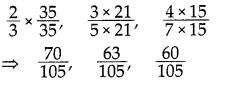Here 60 < 63 < 70
∴ $$\frac{4}{7}<\frac{3}{5}<\frac{2}{3}$$

Question 6.
Write the following rational numbers in descending order.
$$\frac{1}{2}, \frac{1}{3}, \frac{1}{5}, \frac{1}{7}$$
$$\frac{1}{2}, \frac{1}{3}, \frac{1}{5}, \frac{1}{7}$$
L.C.M. of 2, 3, 5 and 7 = 210
Now making the denominators same, we get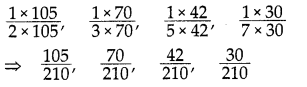Here 105 > 70 > 42 > 30
∴ $$\frac{1}{2}>\frac{1}{3}>\frac{1}{5}>\frac{1}{7}$$

Question 7.
Compare the following rational numbers.
(i) $$\frac{2}{7}$$ and $$\frac{5}{9}$$
latex]\frac{2}{7}[/latex] and $$\frac{5}{9}$$ = $$\frac{2 \times 9}{7 \times 9}$$
and $$\frac{5 \times 7}{9 \times 7}=\frac{18}{63}<\frac{35}{63}$$
∴ $$\frac{2}{7}<\frac{5}{9}$$

(ii) $$\frac{3}{5}$$ and $$\frac{4}{9}$$
$$\frac{3}{5}$$ and $$\frac{4}{9}$$ = $$\frac{3 \times 9}{5 \times 9}$$
and $$\frac{4 \times 5}{9 \times 5}=\frac{27}{45}>\frac{20}{45}$$
∴ $$\frac{3}{5}>\frac{4}{9}$$

Question 8.
Fill in the blanks: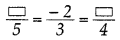Let us fill up the blanks with x and y as follows:
$$\frac{x}{5}=\frac{-2}{3}=\frac{y}{4}$$
⇒ $$\frac{x}{5}=\frac{-2}{3}$$ and $$\frac{-2}{3}=\frac{y}{4}$$
⇒ 3x = -10 and 3y = -8
⇒ x = $$\frac{-10}{3}$$
and y = $$\frac{-8}{3}$$

Question 9.
Find the absolute values of the following rational numbers.
$$\frac{-5}{7}, \frac{0}{-5}, \frac{-3}{-4}$$
Absolute value of $$\frac{-5}{7}=\left|\frac{-5}{7}\right|=\frac{5}{7}$$
Absolute value of $$\frac{0}{-5}=\left|\frac{0}{-5}\right|=\frac{0}{5}$$ = 0
Absolute value of $$\frac{-3}{-4}=\left|\frac{-3}{-4}\right|=\frac{3}{4}$$Question 10.
Represent the following rational numbers on the same number line.
(i) $$\frac{-3}{2}$$
(ii) $$\frac{3}{5}$$
(iii) $$\frac{-3}{5}$$
(iv) $$\frac{3}{2}$$
(v) $$\frac{-2}{3}$$